﻿ Using Multivariant Regression Analysis to Compute Longevity Risk and Actuarial Liability: The Case of Social Benefits for Workers in VenezuelaPublications are Open
Access in this journal
Article Versions
Export Article
• Normal Style
• MLA Style
• APA Style
• Chicago Style
Research Article
Open Access Peer-reviewed

Using Multivariant Regression Analysis to Compute Longevity Risk and Actuarial Liability: The Case of Social Benefits for Workers in Venezuela

Evaristo Diz, J. Tim Query
Journal of Finance and Economics. 2018, 6(4), 144-153. DOI: 10.12691/jfe-6-4-4
Published online: August 06, 2018

Abstract

In this study, a multivariate regression model is determined that allows computation of the actuarial liability of social benefits using a group of potential predictors. In general, the previous or independent predictors are the same as those utilized in an actuarial valuation of labor commitments. Several linear and non-linear models are considered and tested in this study. Among the most important findings of this research is that the PBO or Actuarial Liability depends fundamentally on a linear basis of two fundamental variables in the quantification of Social Benefits -- the Guarantees and the Social Benefits to Pay (PSP). The attractiveness of a relatively simple yet structurally robust model is a very attractive option in situations where managers are tasked to provide estimates in relatively short order, given that the two variables highlighted are generally readily available for most organizations. Realistically, companies are often required to give quick estimates, and they find it quite difficult to quantify an order of this magnitude. This model succeeds in filling this informational gap at the 4 percent interest rate.

1. Introduction

Longevity risk, as applied to pension funds or life insurance annuities, is the risk exposure of higher-than-expected payout ratios. Longevity risk exists due to the increasing life expectancy trends among policyholders and pensioners, and can result in payout levels that are higher than originally projected by a company. The types of plans exposed to the greatest levels of longevity risk are defined-benefit pension plans and annuities, which guarantee lifetime benefits for policy or plan holders.

Calculating future liability of social benefits is an increasingly important issue, in Venezuela and all over the world. The world’s six largest pension saving systems – the United States, United Kingdom, Japan, Netherlands, Canada and Australia – are expecting a funding shortfall of \$224 trillion by 2050. If China and India are included, which have the world’s largest populations, the combined savings gap for the eight countries reaches a total of \$400 trillion by 2050, a sum equivalent to five times the size of the current global economy 1.

The situation is especially dire in Venezuela. Venezuela’s economy is built on oil—its leaders boast it has the world’s largest proven reserves—and it is tempting to blame fickle crude prices for its woes. Venezuela has lost half of its economy since 2013. However, the problem extends beyond oil and it’s getting worse. Unemployment will reach 30% and prices on all types of goods in the country will rise 13,000% this year, according to figures published by the International Monetary Fund 2.

Pensions in Venezuela pay very little: 177,000 bolivares a month, or about \$5 on the black market, while a kilo of flour, rice or grain can easily cost up to 30,000 bolivares 3.

In this study, we determine a multivariate regression model that allows computation of the actuarial liability of social benefits using a group of potential predictors. In general, the previous or independent predictors are the same as those utilized in an actuarial valuation of labor commitments.

Creating a relevant and reasonably accurate Multivariate Regression Model would provide management timely estimates of actuarial liability levels by management for company employees entitled to Social Benefits. This would be a more efficient and economical alternative than engaging in a formal actuarial valuation. Obviously, as with any model, there is an estimation error at a predetermined confidence level. However, that error is not materially important in terms of the order of magnitude.

The rest of this paper is organized as follows. A brief review of relevant literature in the next section will be followed by a description of the methodology. A discussion of the results and their implications follows, with the conclusion closing the paper.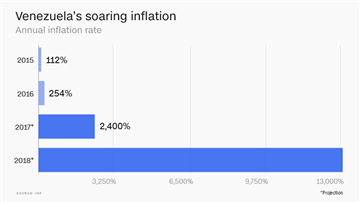2. Literature Review

As pointed out by 4, the effort to model mortality dependence for multiple populations has important implications for mortality/longevity risk management. Their study presents a dynamic multi-population motality analysis using copula models to assess multivariate dependence. Their simple and flexible, model in terms of model specification, is widely applicable to high dimension data.

The financial implications of ageing populations globally have garnered increased attention regarding the complex modeling of trends and volatility related to longevity risk. Financial models that allow for risk pricing and risk management have attracted increasing attention along with multiple factor models. A study by 5 investigates common trends through co-integration, and the factors driving the volatility of mortality using principal components analysis for many developed countries. They find the need for multiple factors when modeling mortality rates across all these countries, and that trends by countries are stochastic. They posit that the basic structure of the Lee-Carter model inadequately models the random variation and the full risk structure of mortality changes.

For organizations facing changes in a social benefit system that mandates employer contributions, a major problem facing decision-makers is forecasting the impact of those changes for companies in different sectors of the economy. The processing of information on an individualized basis can be nearly impossible when facing time constraints, making the development of a model imperative. 6 tackle the issues raised above by identifying key variables. The impact is explained using a reduced multivariable statistical model. This study uses a proprietary data set to model a methodology for measuring the rigor of these regulatory changes. Three key explanatory variables – the number of employees; the cumulative warranty; the monthly average full salary – are analyzed to determine the impact on those companies. Among these, the most important variable and in terms of statistical significance is the amount of accumulated warranty at five percent, followed by the salary and the number of employees in the company, respectively.

Multivariate models are also used to value longevity risk premiums and can confirming that longevity-linked securities offer inexpensive opportunities for asset managers. 7 extends the standard Campbell-Viceira strategic asset allocation model by including a longevity-linked investment possibility. Their model estimation demonstrates that aggregate shocks to survival probabilities are reliable forecasters for long-term returns of longevity-linked securities.

3. Methodology

3.1. Description of the Multivariate Regression Model

To carry out the study’s objectives, we begin with a proprietary database of 53 companies, comprised of a variety of firms representing different sizes, with diverse demographic variables and also with dissimilar liabilities, comparable to what we would expect to find in the real world. The filter for selection was that Pension Benefit Obligations (PBO) were determined at the same year of data, using 4 percent as the real rate of interest. From the previous date, a correlation analysis was performed to obtain the degree of association between the variables in order to rule out collinearity between them.

The variables used in the model are as follows:

1. Age: Average age of company employees

2. Guarantee: Social benefits accumulated by guarantee for the total employees

3. Number: Total number of employees

4. PBO (Pension Benefit Obligation): Actuarial Liability for Benefits

5. Benefits: Social benefits

6. Salary: Average Integral Salary of Employees

7. Service: Average Age

• Chart 1. Correlation Matrix

Structure of the Data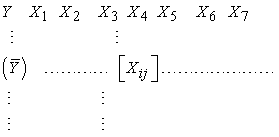Vector Associated with PBO Dependent Variable

Independent Variables Matrix.

3.2. Multivariate Analysis

Data / Variables:

SENIORITY

AGE

EMPLOYEES

WARRANTY

PBO

PSP (SOCIAL BENEFITS PAYABLE)

SALARY

There are 53 complete cases used in the calculations. This procedure is designed to summarize several columns of quantitative data. It will calculate several statistics, including correlations, covariances and partial correlations. Also included in the procedure are a series of multivariate graphs, which provide interesting observations of the data. From these results, a statistical model of the data will be constructed. The objective is to construct a model to predict the PBO given the values of one or more independent variables.

Table 1 below provides the statistical summary for each of the selected variables. It includes measures of central tendency, variability, and form. Of particular interest here is standardized bias and standardized kurtosis, which can be used to determine if the sample comes from a normal distribution.

3.3. Correlations

In Table 2 we can observe the Pearson product moment correlations, between each pair of variables. The range of these correlation coefficients ranges from -1 to +1, and they measure the strength of the linear relationship between the variables. The number of data pairings used to calculate each coefficient is also shown in brackets.

The third number in each block of the table is a P-value that tests the statistical significance of the estimated correlations. P-values below 0.05 indicate correlations significantly different from zero, with a confidence level of 95.0%. The following pairs of variables have P-values below 0.05: SENORITY and AGE

SENORITYand GUARANTEE

SENORITYand PBO

SENORITYand PSP

AGE and SALARY

EMPLOYEES AND GUARANTEE

EMPLOYEES and PBO

EMPLOYEES and PSP

GUARANTEE and PBO

GUARANTEE and PSP PBO and SP

3.4. Selection of Regression Model - PBO

In order to select the best multivariate model that explains the association of PBO with one or more predictor variables, the different combinations of the dependent variable are run with all the independent variables.

Dependent variable: PBO

Independent variables:

A = SENIORITY

B = AGE

C = EMPLOYEES

D = WARRANTY

E = PSP

F = SALARY

Number of completed cases: 53

The results of fitting multiple regression models to describe the relationship between PBO and 6 predictor variables is illustrated in Table 3. The models have been adjusted containing all combinations from 0 to 5 variables. Tabulated statistics include mean error square (CME), adjusted and unadjusted R-Square values, and the Mallows Cp statistic.{1} To determine which models are best according to these different criteria.

4. Results

In this section, we present and analyze our results. The results of fitting multiple regression models to describe the relationship between PBO and 6 predictor variables is illustrated in Table 3. The models have been adjusted containing all combinations from 0 to 5 variables. Tabulated statistics include mean error square, adjusted and unadjusted R-Square values, and the Mallows Cp statistic. These measures are used to help determine which models are best according to these different criteria.

Table 4 displays the models that give the highest values of adjusted R-squared. The adjusted R-squared statistic measures the proportion of variability in PBO that is explained by the model. Large values of adjusted R-squares correspond to small mean square error values. Up to 5 models are shown in each subset of 0 to 5 variables. The best model contains 5 variables, SENIORITY, AGE, GUARANTEE, PSP, and SALARY.

• Graphic 2. Adjusted R2 for pension benefit obligation
• Graphic 3. Mallow's Cp for the benefit of pension Obligations

Table 5 below shows the models that give the smaller values of the statistical Cp of Mallows. Cp is a measure of bias in the model, based on the comparison between the mean square of the total error and the variance of the true error. Non-bias models have an expected value of about p, where p is the number of coefficients in the adjusted model (including the constant). You should look for models with Cp values close to p.

• Graphic 4. The square of the average errors for the benefit of pension obligations
• Graphic 5. R-square for pension commitments
4.1. Multiple Regression - PBO

Based on the previous selection we proceeded to use the model with two independent variables having the lower Cp and also utilized the principle of parsimony regarding the smallest number of possible variables.

Dependent variable: PBO

Independent variables:

PSP

WARRANTY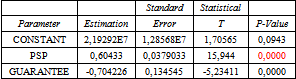5.1. Analysis of Variance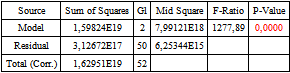R-square = 98.0812 percent

R-square (adjusted for g.l.) = 98.0044 percent

Standard error of est. = 7.90787E7

Mean Absolute Error = 3.66167E7

Durbin-Watson Statistic = 1.97114 (P = 0.4729)

Autocorrelation of residuals in delay 1 = 0.0136931

The output shows the results of fitting a multiple linear regression model to describe the relationship between PBO and 2 independent variables. The equation of the fitted model is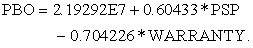Since the P-value in the ANOVA table is less than 0.05, there is a statistically significant relationship between the variables with a confidence level of 95.0%. The R-Squared statistic indicates that the adjusted model explains 98.0812% of the variability in PBO. The adjusted R-Squared statistic, which is more appropriate to compare models with different number of independent variables, is 98.0044%. The standard error of the estimate shows that the standard deviation of the residuals is 7.90787E7. This value can be used to construct limits for new observations by selecting the Reports option from the text menu. The mean absolute error (MAE) of 3.66167E7 is the average value of the waste. The Durbin-Watson (DW) statistic examines the residuals to determine if there is any significant correlation based on the order in which they are presented in the data file. Since the P-value is greater than 0.05, there is no indication of a serial autocorrelation in the residues with a confidence level of 95.0%.

To determine if the model can be simplified, note that the highest P-value of the independent variables is 0.0000, which corresponds to GUARANTEE. Since the P-value is less than 0.05, that term is statistically significant with a confidence level of 95.0%. Consequently, we probably should not remove any variables from the model.

• Graph 7. Graphic Component Residual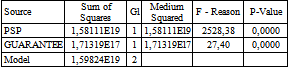This table shows the statistical significance of each variable as it was added to the model. You can use this table to help you determine if the model can be simplified, especially if you are adjusting a polynomial. In sample how the relation is linear or polynomial of degree 1.

5.3. 95.0 Percent Confidence Intervals for Coefficient EstimatesThis table shows 95.0% confidence intervals for the coefficients in the model. The confidence intervals show how accurately the coefficients can be estimated given the amount of data available, and the level of noise that is present.

This table shows the estimated correlations between the coefficients in the adjusted model. These correlations are used to detect the presence of severe multicollinearity, that is, correlation between the predictor variables. In this case, there is 1 correlation with absolute value greater than 0.5 (not including the constant term).

5.4. Atypical Residues

This table contains information about PBO, which is generated using the adjusted model. The table includes:

(1) predicted PBO values using the adjusted model

(2) the standard error for each predicted value

(3) 95.0% forecast intervals for new observations

(4) 95.0% confidence intervals for the mean response

The table of atypical residues lists all the observations that have Residues greater than 2, in absolute value. The Remaining residues measure how many standard deviations deviate each observed PBO value from the adjusted model, using all data except that observation. In this case, there are 3 researched residues greater than 2, 2 greater than 3. It is convenient to examine the observations with residues greater than 3 to determine if they are aberrant values that should be removed from the model and treated separately. In fact, only 2 out of 53 observations would be within the classification of atypical residues in our literal opinion and the fundamental reason is that these two companies are significantly different from the rest.

5.5. Chain Regression - PBO

This procedure is designed to provide estimates of regression coefficients when independent variables are strongly correlated. By allowing a slight amount of bias (bias), the accuracy of the estimates can often increase greatly. In this case, the adjusted regression model is

Dependent variable: PBO

Independent variables:

PSP

WARRANTY

Number of completed cases: 53

R-Square = 98.0812 percent

R-Square (adjusted by g.l.) = 98.0044 percent

Standard error of est. = 7.90787E7

Absolute mean error = 3.66167E7

Durbin-Watson Statistic = 1.97114

Residual delay autocorrelation 1 = 0.0136931.The current value of the string parameter is 0.0, which is equivalent to ordinary least squares. The string parameter is normally set between 0 and 1. To determine a good value for the string parameter, you should examine the standardized regression coefficients or the variance inflation factors.

The R-Squared statistic indicates that the model, thus adjusted, explains 98.0812% of the variability in PBO. The adjusted R-Squared statistic, which is more suitable for comparing models with different number of independent variables, is 98.0044%. The standard error of the estimate shows that the standard deviation of the residuals is 7.90787E7. The mean absolute error (MAE) of 3.66167E7 is the average value of the waste. The Durbin-Watson (DW) statistic tests the residuals to determine if there is any significant correlation based on the order in which they were presented in their data file.

This table shows the estimated regression coefficients for chain parameter values between 0.0 and 0.1. As the string parameter is incremented from 0, the coefficients often change dramatically at first, but then become relatively stable. A good value for the string parameter is the smallest value after which the estimates change slowly.

Table 12 shows the standardized regression coefficients for regression parameter values between 0.0 and 0.1. These are the coefficients of the regression model when the variables are expressed in standardized form. As the string parameter is incremented from 0, the coefficients often change dramatically at first, but then become relatively stable. A good value for the string parameter is the smallest value after which the estimates change slowly. It is recognized that this is very subjective, but the chain trace can help you make a good choice.

The model finally chosen is:

Results of the Model for the Chain Parameter = 0.09R-Square = 90.546 percent

R-Square (adjusted by g.l.) = 90.1679 percent

Standard error of est. = 1.25531 E8

Absolute mean error = 6.29326E7

Durbin-Watson Statistic = 2.22691

Residual delay autocorrelation 1 = -0.1113716

This model is designed to provide estimates of regression coefficients when independent variables are strongly correlated. By allowing a slight amount of bias (bias), the accuracy of the estimates can often be greatly increased. In this case, the adjusted regression model isThe current value of the string parameter is 0.09. The string parameter is normally set between 0 and 1. To determine a good value for the string parameter, you should examine the standardized regression coefficients or the variance inflation factors. Generally, this model unlike the previous one, could be more stable in predictions even beyond the domain of the data.

The R-Squared statistic indicates that the model, thus adjusted, explains 90.546% of the variability in PBO. The adjusted R-Squared statistic, which is more adequate to compare models with different number of independent variables, is 90.1699%. The standard error of the estimate shows that the standard deviation of the residues is 1.25531E8. The mean absolute error (MAE) of 6.29326E7 is the average value of the waste.

In many cases, the estimates of the coefficients will change dramatically as it moves away from 0. Eventually, the coefficients will stabilize as they do in the neighborhood of = 0.09. Unfortunately, they no longer apply statistical significance tests, so it is difficult to determine which coefficients are statistically significant. However, it is likely that the highlighting model is much more stable than the ordinary least squares model, particularly when extrapolated outside the experimental region.

6. Conclusion

It is important to note that Actuarial Liabilities, as is well known, depend on a series of financial and demographic assumptions. Several linear and non-linear models are considered and tested in this study. Among the most important findings of this research is that the PBO or Actuarial Liability depends fundamentally on a linear basis of two fundamental variables in the quantification of Social Benefits -- the Guarantees and the Social Benefits to Pay (PSP).

The level of contribution to the determination of the PBO is the guarantee (what the company has already accumulated for the payment). This level of contribution is in the order of 39.42% and the part relative to PSP Social Benefits to be paid is 27.71% with a statistic of adjusted-R squared of 90.16%. It appears only two predictive variables that the model explains reasonably -- the corresponding actuarial liability for the differential of benefits.

Realistically, companies are often required to give quick estimates, and they find it quite difficult to quantify an order of this magnitude. This model succeeds in filling this informational gap at the 4 percent interest rate. Areas of future research include repeating the study with other real rates to see if there is structural stability of the model. The potential of a structural robust model is a very attractive option in situations where managers are tasked to provide estimates in relatively short order, given that the two variables highlighted are generally readily available for most organizations.

Note

1. Mallow's Cp is used to assess the fit of a regression model that has been estimated using ordinary least squares. It is applied in the context of model selection, where many predictor variables are available for predicting some outcome, and the goal is to find the best model involving a subset of these predictors. A small value of Cp means that the model is relatively precise.

References

  World Economic Forum, “We’ll Live to 100 – How Can We Afford It?” In article  Gillespie, Patrick, “Half the Venezuelan Economy has Disappeared.” @CNNMoney. Accessed April 12, 2018. http://money.cnn.com/2018/01/25/news/economy/venezuela-imf/index.html. In article View Article  Alex, G.O., “Government Pensions in Venezuela Used to Mean Security in Old Age — but not any more,” Miami Herald, November 4, 2017. In article  Chen, H., MacMinn, R. D. and Sun, T.017), “Mortality Dependence and Longevity Bond and Insurance. Pricing: A Dynamic Factor Copula Mortality Model With the GAS Structure,” Journal of Risk and Insurance, 84: 393-415. 2017. In article View Article  Njenga, C. & Sherris, M., “Longevity Risk and the Econometric Analysis of Mortality Trends and Volatility.” Asia-Pacific Journal of Risk and Insurance, 5:35-55. 2011. In article View Article  Query, J.T. and E. Diz Cruz, “A Retroactive Impact Analysis of Changes in Social Benefits: The Case of Venezuela, International Journal of Business and Social Research, 5:87-104. 2015 In article View Article  Bisetti, E., Favero, G.N., and Tebaldi, C., “A Multivariate Model of Strategic Asset Allocation with Longevity Risk,” Journal of Financial and Quantitative Analysis, 52: 2251-2275. 2017. In article View Article  Brooks, S. (2007). Globalization and Pension Reform in Latin America. Latin American Politics and Society, 49, 31-62. In article View Article

Published with license by Science and Education Publishing, Copyright © 2018 Evaristo Diz and J. Tim QueryThis work is licensed under a Creative Commons Attribution 4.0 International License. To view a copy of this license, visit http://creativecommons.org/licenses/by/4.0/